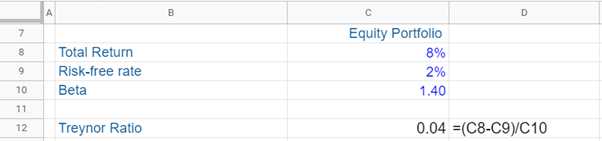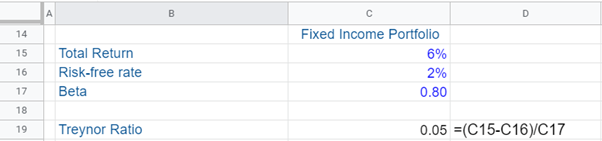## What is “Treynor Ratio?”

The Treynor ratio, a part of the Capital Asset Pricing Model (CAPM), measures a portfolio manager’s returns in excess of the risk-free rate, while also factoring in risk. Basically, this ratio is an investment portfolio performance measure. However, unlike the Sharpe ratio, the Treynor ratio compares the excess returns to only systematic risk, rather than using the standard deviations of returns as a measure of risk. So, the Treynor ratio is a return-to-risk ratio which uses beta – a measure of systematic risk and calculates the extent to which a portfolio or stock correlates or moves along with the broad market.

A stock or portfolio that has a beta of greater than 1 is riskier than a security or portfolio that is less than one. This again is a measure of systematic risk and it is risk at a macro level that cannot be diversified away by the portfolio manager. So, the beta is essentially a measure of unavoidable risk.

## Key Learning Points

• For a diversified portfolio, the Treynor ratio is thought of as the best performance measure;
• The higher the Treynor ratio, the better the risk-adjusted return i.e. a higher return per unit of systematic risk; and
• For portfolios that are not fully diversified, the Sharpe ratio is a more appropriate measure than the Treynor ratio.

## Treynor Ratio, Importance, Formula and Disadvantages

Some portfolio managers and analysts prefer the Treynor ratio over the Sharpe ratio, when they are looking at diversified portfolios. This is because in such portfolios only systematic risk is remaining as all other risks are diversified away. This systematic risk always persists, because of volatile behavior of financial markets.

The Treynor ratio is thought of the best performance measure for a diversified portfolio and is used to analyze and manage the systematic risk. Further, this ratio is helpful vis-à-vis managing investment decisions to improve and optimize portfolio returns.

Formula:

Treynor Ratio = (Rp – rf)/ βp

Rp – rf = Excess returns (i.e. return on a portfolio minus the risk-free rate)

Rp = Portfolio return

Rf = Risk-free rate (return on government securities, for example Treasury Bills)

Βp = beta of the portfolio (i.e. measure of systematic or non-diversifiable risk)

This formula reflects that the Treynor ratio is concerned with both portfolio return and its systematic risk, and shows excess returns per unit of systematic risk. The higher the Treynor ratio, the better the risk-adjusted return i.e. a higher return per unit of systematic risk and consequently a better performance of the portfolio manager.

The Treynor ratio, besides other ratios such as the Sharpe ratio etc. are very important for an investment management firm, as the they concerned with investment returns and also how the fund managers have performed relative to a relevant benchmark (stock market index, for example, the S&P 500 in the US) and their peers.

It’s important to note that the Treynor ratio does not provide meaningful values for negative values of beta. Further, though the Treynor ratio can be used to rank investment portfolios, it does not provide information on whether these investment portfolios are superior or better than the market portfolio.

For portfolios that are not fully diversified, what matters is the total risk, rather than the systematic risk alone. In such cases, the Sharpe ratio is a more appropriate performance measure than the Treynor ratio.

## Treynor Ratio, Example

Given below are two portfolios, and we use the Treynor Ratio to decide which portfolio is a better investment. The first portfolio is an equity portfolio and the other is a fixed income portfolio.

Assume that total return on the equity portfolio and fixed income portfolio is 8% and 6% respectively. Further, the risk-free rate is 2%. Moreover, we assume that the beta on the equity portfolio and fixed income portfolio is 1.40 and 0.8 respectively. Using this information, we compute the Treynor ratio for both portfolios.The Fixed Income portfolio has a higher Treynor ratio, which suggests that this portfolio has a more favorable risk/return mix than the fixed income portfolio. Therefore, it is advisable for an investor to invest in the Fixed Income portfolio.